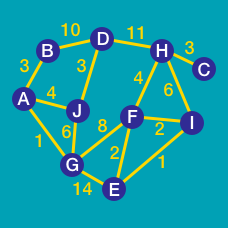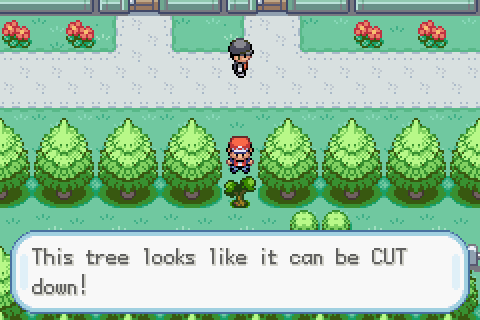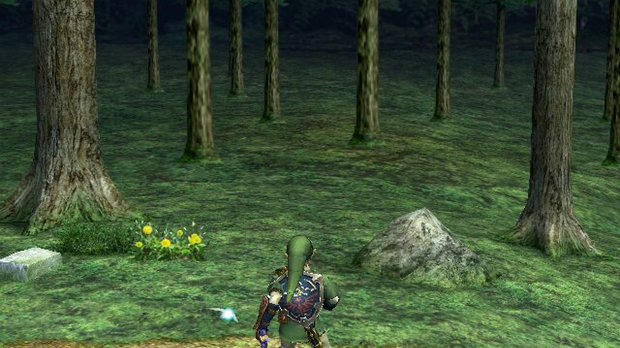Computer Science

# Graph Algorithms: Level 4 ChallengesThe forest is getting thicker, darker, and more dangerous. Heart Pieces are getting rarer, and open ground is no longer as abundant. Our hero now faces thick, but removable Trees in addition to the impenetrable ones, and while they are manageable, clearing Trees requires a fair bit of magic and work, so Link would prefer to cut as few Trees as possible.

This link (1) contains a zip file, with a single file in the Input Format specified for this collection of problems, of 1000 mazes.

Let $$S$$ be the sum of the score for each maze. We define the score to be the index of the maze (1-based) multiplied by the minimum number of Trees Link must cut in order to reach the singular Heart Piece in the maze. If Link can reach the Heart Piece without cutting any Trees, the score is zero for that maze. Link will always be able to reach the Heart Piece somehow, however.

Find $$\boxed{S}$$.

Example:

Input:

3
3 3
L..
T#.
H..
5 5
L....
T###.
T###T
H###.
.....
10 1
LTTTTTTTTH


Output:

26


Output Explained:

In the first maze, the Heart Piece is very close by if Link cuts the Tree, but if he takes the long way, he doesn't need to cut any, so the score is $$0$$ .

Likewise, in the second maze, the Heart Piece is very close by if Link cuts the two Trees below, but if he takes the long way, he only needs to cut one Tree, so the score is $$2 \times 1$$ .

In the third maze, Link has only one option, to cut the 8 trees in between him and the Heart Piece, so the score is $$3 \times 8$$ .

$$0 + 2 \times 1 + 3 \times 8 = 26$$

Details and Assumptions:

• Link will always appear once per maze, as an 'L'.
• There will be exactly one Heart Piece, as 'H', and it will be accessible.
• Open ground is given as '.'.
• Impenetrable trees are given as '#'.
• Cuttable trees are given as 'T'.
• No other characters will be present in each maze.
• $$1 \leq W,H \leq 100$$ in all mazes.

(1) - This link currently points to my Dropbox account, since there is currently no way to upload non-image content to Brilliant. Admin, feel free to delete this footnote if this is changed.

###### Image Credit: https://www.kirsle.net/firered/full

There are nine clocks on a $$3 \times 3$$ array labeled A to I.The goal is to return all the dials to 12 o'clock with as few moves as possible.There are nine different allowed ways to turn the dials on the clocks. Each such way is called a move. Select for each move a number 1 to 9. That number will turn the dials 90' (degrees) clockwise on those clocks which are affected according to the table below.

 Move Affected clocks 1 ABDE 2 ABC 3 BCEF 4 ADG 5 BDEFH 6 CFI 7 DEGH 8 GHI 9 EFHI

For the configuration in the picture above, let $$L$$ be the shortest possible sequence of moves that will lead to all the clocks being $$12$$ o'clock. Off all possible values of $$L$$,what is the smallest prime value of $$L$$?

Explanatory examples

• We do not care about the clocks minute hand in this problem. A clock at 6 after one move(rotated clock wise) becomes 9 and a clock at 12 after one move becomes 3 and so on.

• If the sequence of moves had been $$L=$$ $$1$$ and $$3$$, the answer would have been $$13$$ instead of $$31$$ because it is the smallest prime value of $$L$$.Our hero, Link, finds himself in a forest most plain. Scattered around him, northways, eastways, westways and southways, are open plots of grass, impenetrable woods, and scattered, rare Heart Pieces. This time, we wonder if Link can get just one of these Heart Pieces, and if so, how many steps he needs to take to reach it.

This link (1) contains a zip file, with a single file in the Input Format specified for this collection of problems, of $$1000$$ mazes.

Let $$S$$ be the sum of the score for each maze. We define the score to be the index of the maze (1-based) multiplied by the minimum number of steps Link must take to reach a single Heart Piece, if he can. If no Heart Piece can be reached, the score for that maze is zero.

Find $$\boxed{S}$$ .

Example:

Input:

3
6 1
H.L..H
2 2
L#
#H
5 5
L....
####.
..H#.
.###.
.....


Output:

50


Output Explained:

In the first maze, there is are two Heart Pieces, but the one on the left is closer, at $$2$$ steps away instead of $$3$$, so the score for the maze is $$1$$ (the index) times $$2$$.

In the second maze, there is a single Heart Piece, but it cannot be accessed, so the score for that maze is zero.

In the third maze, there is a single Heart Piece, and it is reachable in precisely $$16$$ steps, so the score for this maze is $$48$$.

$$2 + 48 = \boxed{50}$$

Details:

• Link will always appear once per maze, as an 'L'.
• There will be a positive number of Heart Pieces per maze, as 'H'.
• Open ground is given as '.'.
• Impenetrable trees are given as '#'.
• No other characters will be present in each maze.
• $$1 \leq W,H \leq 100$$ in all mazes.

(1) - This link currently points to my Dropbox account, since there is currently no way to upload non-image content to Brilliant. Admin, feel free to delete this footnote if this is changed.